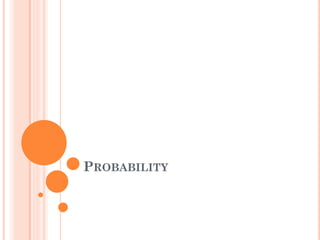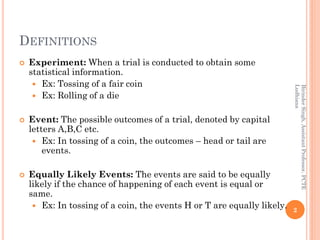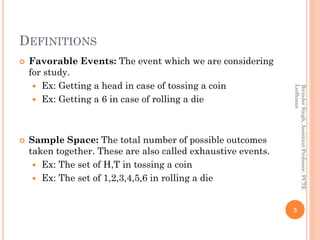Diese Präsentation wurde erfolgreich gemeldet.

# Probability - I×

1 von 24
1 von 24

# Probability - I

This presentation includes the concepts along with concrete examples, definitions, problems of addition, multiplication and bayes' theorem.

This presentation includes the concepts along with concrete examples, definitions, problems of addition, multiplication and bayes' theorem.

### Probability - I

1. 1. PROBABILITY
2. 2. DEFINITIONS  Experiment: When a trial is conducted to obtain some statistical information.  Ex: Tossing of a fair coin  Ex: Rolling of a die  Event: The possible outcomes of a trial, denoted by capital letters A,B,C etc.  Ex: In tossing of a coin, the outcomes – head or tail are events.  Equally Likely Events: The events are said to be equally likely if the chance of happening of each event is equal or same.  Ex: In tossing of a coin, the events H or T are equally likely. 2 BirinderSingh,AssistantProfessor,PCTE Ludhiana
3. 3. DEFINITIONS  Favorable Events: The event which we are considering for study.  Ex: Getting a head in case of tossing a coin  Ex: Getting a 6 in case of rolling a die  Sample Space: The total number of possible outcomes taken together. These are also called exhaustive events.  Ex: The set of H,T in tossing a coin  Ex: The set of 1,2,3,4,5,6 in rolling a die 3 BirinderSingh,AssistantProfessor,PCTE Ludhiana
4. 4. DEFINITIONS  Complementary Events are two outcomes of an event that are the only two possible outcomes.  Ex: flipping a coin and getting heads or tails. Head is complement to tail.  In rolling of a die, getting 1 or 2 are not complementary events. 4 BirinderSingh,AssistantProfessor,PCTE Ludhiana
5. 5. DEFINITIONS  Mutually Exclusive Event: When two events can’t happen simultaneously in a single trial. In other words, when the happening of one excludes the happening of other.  Ex: In tossing of a coin, events head and tail are MEE. 5 BirinderSingh,AssistantProfessor,PCTE Ludhiana
6. 6. DEFINITIONS  Mutually Inclusive Event: When two events can happen simultaneously in a single trial. In other words, when there is something common in between the two events.  Ex: Getting a red card or a king 6 BirinderSingh,AssistantProfessor,PCTE Ludhiana
7. 7. PROBABILITY  It is the likeliness of any event i.e. what is the chance that particular event will happen?  Eg:  What is the probability that CSK will win IPL 2018?  What is the probability that it will rain today?  What is the probability that after going through these slides you understand Probability? Probability = Favorable outcomes / Total outcomes 7 BirinderSingh,AssistantProfessor,PCTE Ludhiana
8. 8. PRACTICE PROBLEMS Q1: In case of rolling a die, what is the probability of getting: a) an even number b) prime number c) not even number d) Number greater than 4 Q2: In a lottery, there are 10 prizes and 90 blanks. If a person holds one ticket a) an even number b) prime number c) not even number 8 BirinderSingh,AssistantProfessor,PCTE Ludhiana
9. 9. PRACTICE PROBLEMS Q3: In case of drawing a card, what is the probability of getting: a) an ace b) Red card c) Face card d) Black king Q4: What is the probability that a non leap year selected at random will contain 53 Sundays? Q5: What is the probability that a leap year selected at random will contain 53 Sundays? 9 BirinderSingh,AssistantProfessor,PCTE Ludhiana
10. 10. PRACTICE PROBLEMS Q6: Two dice are thrown, what is the probability of getting: a) Sum of 11 b) Sum of 10 10 BirinderSingh,AssistantProfessor,PCTE Ludhiana
11. 11. THEOREMS OF PROBABILITY  Addition Theorem (For Mutually Exclusive & not Mutually Exclusive)  Multiplication Theorem (For Independent Events & Dependent Events) 11 BirinderSingh,AssistantProfessor,PCTE Ludhiana
12. 12. ADDITION THEOREM (+ / U / OR) 12 BirinderSingh,AssistantProfessor,PCTE Ludhiana
13. 13. PRACTICE PROBLEMS Q1: A card is drawn. What is the probability of getting either a king or queen? Q2: A bag contains 30 balls numbered 1 to 30. One ball is drawn at random. Find the probability that the number of the ball is i. a multiple of 5 or 8 ii. a multiple of 3 or 4 Q3: An urn contains 4 red, 5 black, 3 yellow and 11 green balls. A ball is drawn at random. Find the probability that: i. It is either red, black or yellow ball ii. It is either red, black, green or yellow ball 13 BirinderSingh,AssistantProfessor,PCTE Ludhiana
14. 14. PRACTICE PROBLEMS Q4: A card is drawn at random. What is the probability that: i. It is either a king or black card ii. It is either an ace or club 14 BirinderSingh,AssistantProfessor,PCTE Ludhiana
15. 15. DEFINITIONS  15 BirinderSingh,AssistantProfessor,PCTE Ludhiana
16. 16. MULTIPLICATION THEOREM (X / ∩/ AND)  If A and B are two independent events, then the probability of the simultaneous occurrence of A and B is equal to the product of their individual probabilities. i.e.  P (A∩B) = P(A) . P(B)  If A and B are two dependent events, then the probability of the simultaneous occurrence of A and B is equal to the probability of one event multiplied by the conditional probability of the other event. i.e.  P(A∩B) = P(A) . P(B/A) 16 BirinderSingh,AssistantProfessor,PCTE Ludhiana
17. 17. PRACTICE PROBLEMS Q1: A coin is tossed three times. What is the probability of getting all the three heads? (1/8) Q2: From a pack of 52 cards, two cards are drawn at random. What is the probability that both cards are kings if : i. Drawn with replacement (1/169) ii. Drawn without replacement (1/221) Q3: A bag contains 4 red balls, 3 white balls and 5 black balls. Two balls are drawn one after the other. Find the probability that the first is red and the second is black if: i. Drawn with replacement (5/36) ii. Drawn without replacement (5/33) 17 BirinderSingh,AssistantProfessor,PCTE Ludhiana
18. 18. PRACTICE PROBLEMS Q4: An electronic device is made of three components A, B and C. The probability of failure of the component A, B & C is 0.01, 0.02 and 0.05 respectively. Find the probability that the device will work satisfactorily. (0.92) Q5: A person is known to hit the target in 3 out of 4 shots whereas another person is known to hit the target in 2 out of 3 shots. Find the probability of the target being hit at all when both of them try. (11/12) Q6: A problem is given to three students A, B and C whose chance of solving it are 1/2, 1/3 and ¼ respectively. What is the probability that the problem will be solved? (3/4) 18 BirinderSingh,AssistantProfessor,PCTE Ludhiana
19. 19. PRACTICE PROBLEMS Q7: Four cards are drawn without replacement. What is the probability that they are all aces? (1/270725) Q8: A bag contains 5 white balls and 3 red balls, four balls are successively drawn out and not replaced. What is the chance that balls appear alternatively in color? (1/7) Q9: Four cards are drawn without replacement. What is the probability that: i. They are of the same suit (44/4165) ii. They are of different suits (2197/20825) 19 BirinderSingh,AssistantProfessor,PCTE Ludhiana
20. 20. PRACTICE PROBLEMS Q10: A can hit a target 4 times in 5 shots, B 3 times in 4 shots and C twice in 3 shots. What is the probability that at least two shots hit? (5/6) Q11: Three coins are tossed simultaneously. What is the probability that they will fall two heads and one tail? (3/8) Q9: Four cards are drawn without replacement. What is the probability that: i. They are of the same suit (44/4165) ii. They are of different suits (2197/20825) 20 BirinderSingh,AssistantProfessor,PCTE Ludhiana
21. 21. PRACTICE PROBLEMS – BAYES’ THEOREM  An insurance company insured 2000 scooter drivers, 4000 car drivers and 6000 truck drivers. The probability of an accident involving a scooter, a car and a truck are 0.01, 0.03 and 0.15 respectively. One of the insured persons meets with an accident. What is the probability that he is a scooter driver? (1/52)  A factory has two machines A and B. Past record shows that machine A produced 60% of the items of output and machine B produced 40% of the items. Further, 2% of the items produced by machine A and 1% produced by machine B were defective. All the items are put into one stockpile and then one item is chosen at random from this and is found to be defective. What is the probability that it was produced by machine B? (1/4) 21 BirinderSingh,AssistantProfessor,PCTE Ludhiana
22. 22.  A coin is tossed three times, consider the following events. A: ‘No head appears’, B: ‘Exactly one head appears’ and C: ‘Atleast two heads appear’. Find their probabilities.  One card is drawn from a well shuffled deck of 52 cards. If each outcome is equally likely, calculate the probability that the card will be (i) a diamond (ii) not an ace (iii) a black card (iv) not a diamond (v) not a black card.  A bag contains 9 discs of which 4 are red, 3 are blue and 2 are yellow. The discs are similar in shape and size. A disc is drawn at random from the bag. Calculate the probability that it will be (i) red, (ii) yellow, (iii) blue, (iv) not blue, (v) either red or yellow. 22 BirinderSingh,AssistantProfessor,PCTE Ludhiana
23. 23.  Two students Anil and Ashima appeared in an examination. The probability that Anil will qualify the examination is 0.05 and that Ashima will qualify the examination is 0.10. The probability that both will qualify the examination is 0.02. Find the probability that (a) Both Anil and Ashima will not qualify the examination. (b) Atleast one of them will not qualify the examination and (c) Only one of them will qualify the examination. 23 BirinderSingh,AssistantProfessor,PCTE Ludhiana
24. 24.  A letter is chosen at random from the word ‘ASSASSINATION’. Find the probability that letter is (i) a vowel (ii) a consonant  Find the probability that when a hand of 7 cards is drawn from a well shuffled deck of 52 cards, it contains (i) all Kings (ii) 3 Kings (iii) atleast 3 Kings. 24 BirinderSingh,AssistantProfessor,PCTE Ludhiana